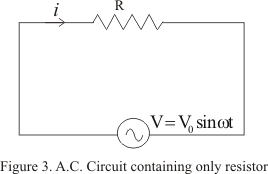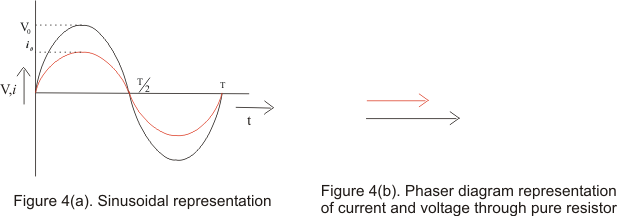# A.C through pure resistor

## A.C through pure resistor

• Figure below shows the circuit containing alternating voltage source V=V0sinω connected to a resistor of resistance R• Let at any instant of time ,i is the current in the circuit ,then from Kirchhoff�s loop rule
V0sinωtRi
or
i=(V0/R)sinωt
=i0sinωt                                                ----(8)
Where,
i0=V0/R                                                ----(9)
• From instantaneous values of alternating voltage and current ,we can conclude that in pure resistor ,the current is always in phase with applied voltage
• Their relationship is graphically represented as